# Percentages + reason - math problems

#### Number of problems found: 86

• On a farm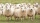On a farm, there are 90 rabbits, 700 sheep, 300 cattle and 500 pigs. What percentage of the total number of animals are rabbits?
• MushroomsFresh mushrooms contain 88% water, dried 14% water. How many kilograms of fresh mushrooms should be collected to get 3 kg of dried?
• Left handedIt is known that 25% of the population is left-handed. What is the probability that there is a maximum of three left-handers at a seminar where there are 30 participants?
• DiscountThe dress was discounted by CZK 115, which was 12% of the original price. What is the cost of the dress after the discount?
• Associative law multiplicationIn a warehouse, you obtain a 20% discount but you must pay a 15% sales tax. Which would you prefer to have calculated first: discount or tax? Explain. (result write as: 1 = first discount, 2 = first tax, 3 = no matter what first)
• Utopia IslandThe probability of disease A on the island of Utopia is 40%. The probability of occurrence among the men of this island, which make up 60% of all the population (the rest are women), is 50%. What is the probability of occurrence of A disease among women o
• Four numbersThe first number is 50% second, the second number is 40% third, the third number is 20% of the fourth. The sum is 396. What are the numbers?
• Test pointsIf you earned 80% of the possible 40 points, how many points did you miss to get 100%?
• Profit per kiloThe trader sells apples at a profit of 20% per kilogram. Today, he decided to reduce the price of apples by 10%. How much profit does the trader have today?
• VAT on booksThe cost of a book in the store is 12.5 euros. How much euros is the VAT of this book? VAT is 10%.
• Kaufland sales-off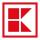As a rule the goods to the approaching end consumption in Kaufland three days before the end of the consumption to 30% off and one day before the end of the consumption to 50% discounts. Sometimes however the seller has been mistaken and the second discou
• Sum on diceWe have two dice. What is the greater likelihood of fall a total sum 7 or 8 ? (write 7, 8 or 0 if the probabilities are the same)?
• Three reds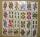What is the probability that when choosing 3 carats from seven carats, all 3 reds will be red?
• Two acesFrom a 32 card box we randomly pick 1 card and then 2 more cards. What is the probability that last two drawn cards are aces?
• Mushrooms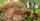Fresh mushrooms contain 90% water, dried only 12%. How many kilograms of fresh mushrooms are needed for 5kg dried?
• One percent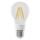One percent of all the lights in the city are LED, the remaining 99% are conventional. Other types are not there. John counted them honestly but he had counted only conventional. After a good dinner he registered numbers and he have notice that from all t
• Unions now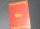The plant has 1,250 employees. The Trade Unions (former ROH, OH) is 18% male, 23% female. Plant management reported that in the trade union is 20% of all employees. How many men and women are in the plant, and how many are men and women in trade unions?
• Summer camp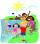Out of the 180 students at a summer camp, 72 signed up for canoeing. Twenty-three students signed up for trekking, and 13 of those students also signed up for canoeing. Use a two-way table to organize the information and answer the following question: App
• ThrowWe throw 2 times with 2 dices. What is the probability that the first roll will fall more than sum of 9 and the second throw have sum 3 or does not have the sum 4?
• Medicine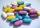We test medicine on 6 patients. For all drug doesn't work. If the drug success rate of 20%, what is the probability that medicine does not work?

Do you have an exciting math question or word problem that you can't solve? Ask a question or post a math problem, and we can try to solve it.

We will send a solution to your e-mail address. Solved examples are also published here. Please enter the e-mail correctly and check whether you don't have a full mailbox.

Our percentage calculator will help you quickly calculate various typical tasks with percentages. Percentages Problems. Reason - math problems.